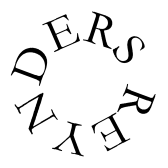From Internet posting:

It's a dununba-type of=20 rhythm.(from Guinea; the Kankan, Kouroussa and Siguiri regions) The dununba's are the dance of the "strong" men. The only dance that is traditionally danced by the Malinke men. There are over 20 different dununba-rhythms. They all orgininate from the Dununb, the mother of all Dununbs.

`12/8       |:  .   .   *   .   .   *   .   .   *   .   .   :|`
`intro      S s S   s   S`
`dundun     .   .   .   .   .   B   B   .   B   B   .   .`
`kenkeni    .   .   B   .   B   B   .   .   B   .   B   B`
`kenbell    .   x   x   .   x   x   .   x   x   .   x   x`
`simplebell x   .   .   x   .   .   x   .   .   x   .   .`
`sangban    B   .   .   M   .   .   B   .   .   M   .   .`
`sangbell   x   .   x   x   .   x   x   .   x   x   .   x`
`dundun         .   .   .   .   B   B   .   B   B   .   .`
`dunbell    x   .   x   x   .   x   x   .   x   x   .   x`

`djembe 1   S   .   O   s   .   .   S   .   O   s   .   .`
`djembe 2   S   .   .   s   O   o   S   .   .   s   O   o`

Solo accompagnement : plays the solo-player when (s)he is not giving accents to a dance but plays something other then the djembes: an example of possibilities:

`solo.acc1  .   .   S   .   O   s   .   .   S   .   O   s`

or (soft played:)

`solo.acc2  S   s   S   s   O   s   S   s   S   s   O   s`

Echauffement: playing more intensely ("warming up the rhythm), getting to the climax of a dance-variation:

`Djem.echf  S   s   S   s   S   s   S   s   S   s   S   o >>`
`           O   s   S   s   S   s   S   s   S   s   S   o (3x)`
`           O   s   S   s   S   o   O   s   S   s   S   o`
`           O   s   S   s   S   o   O   s   S   s   S   .`
`Dun.echf   .   .   .   .   .   B   B   .   B   .   B   B >>`
`bell       x   .   x   x   .   x   x   .   x   .   x   x >>`
`           B   B   .   B   B   B   .   B   B   .   B   B >>`
`           x   x   .   x   x   x   .   x   x   .   x   x >>`
`repeat     .   B   B   .   B   B   .   B   B   .   B   B`
`till      .   x   x   .   x   x   .   x   x   .   x   x`

(Sangban and Kenkeni stay as they are)

Landing : climax, and returning to normal rhitme ; a new dancer or an other dance-variation comes.

`dun.land   .   B   .   B   .   B   .   B   B   .   B   .`
`           .   x   .   x   .   x   .   x   x   .   x   .`
`djem.land  S s S   s   S   .   O o O   o   O   .   Sf  .`